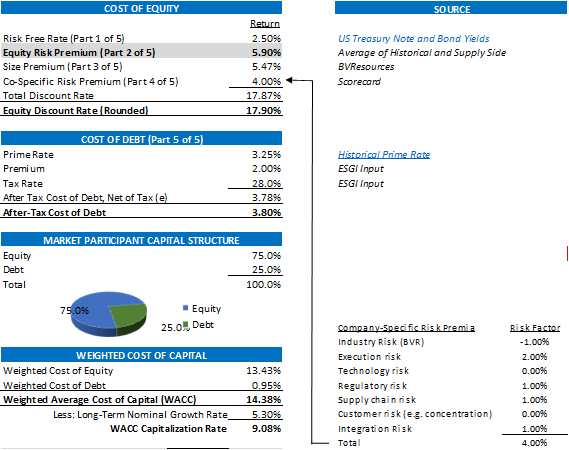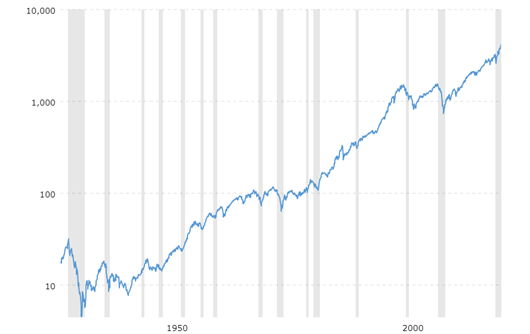# Understanding Discount Rates The Equity Risk Premium – Part 2 of 5

Our prior post and educational discussion of the discount rate as “one of the most important inputs surrounding the valuation of the business” introduced the first input of the build-up approach, the risk-free rate. The second key input is the equity risk premium. In our reports, we define this input as “The ERP represents the extra yields demanded and earned (and risk assumed) over time by equity investments in large public companies over U.S. Treasuries. This premium is sometimes referred to as the market risk premium. It is a measure of systematic risk of equity securities.”

Build-Up Approach – Equity Risk Premium (“ERP”)

Again, in determining the cost of equity, we use the build-up method which starts with a risk-free rate and adds risk components appropriate to the Company to arrive at a total discount rate. A highlight of how we build up both the cost of equity and the weighted cost of capital is pictured below. As noted, the highlighted;Basic Definitions

As shown we are using an ERP of 5.90% which is calculated as follows; “using the S&P 500 average annual return of 11.81% derived from CRSP data for the 1928 – 2020 period and a 5.91% 20-year T-Bond average annual return for the same timeframe.” Said another way, the ERP is difference between a long-term rate of return of a portfolio of equity securities (SP = S&P 500 over the last 72 tears) and a similar long-term risk free rate (RFR = T-Bond average annual return). 

ERP = SP – RFR = 11.81% – 5.91% = 5.90%

Investopedia sums up this concept with the following key take-aways;

1)     The equity-risk premium predicts how much a stock will outperform risk-free investments over the long term.
2)     Calculating the risk premium can be done by taking the estimated expected returns on stocks and subtracting them from the estimated expected return on risk-free bonds.
3)     Estimating future stock returns is difficult, but can be done through an earnings-based or dividend-based approach.

Below is a graphical depiction of the S&P 500 Index from 1928 through 2021 that includes dividends and supports the 11.81% return.What Does This All Mean?

The discount rate is a simple build-up of risk. When valuing the equity of a privately held company, the starting point for this calculation is always a risk-free rate which represents a risk free debt security with little to no risk of default. Because the scope of work is the value of equity of a private equity security, we need to build up this rate with the risk associated with equity.

Stay tuned for Part 3 of 5, the Size Premium where the difference in risk between and small companies.

Exit Strategies values control and minority ownership interests of private businesses for tax, financial reporting, strategic purposes. If you’d like help in this regard or have any related questions, you can reach Joe Orlando, ASA at 503-925-5510 or jorlando@exitstrategiesgroup.com.